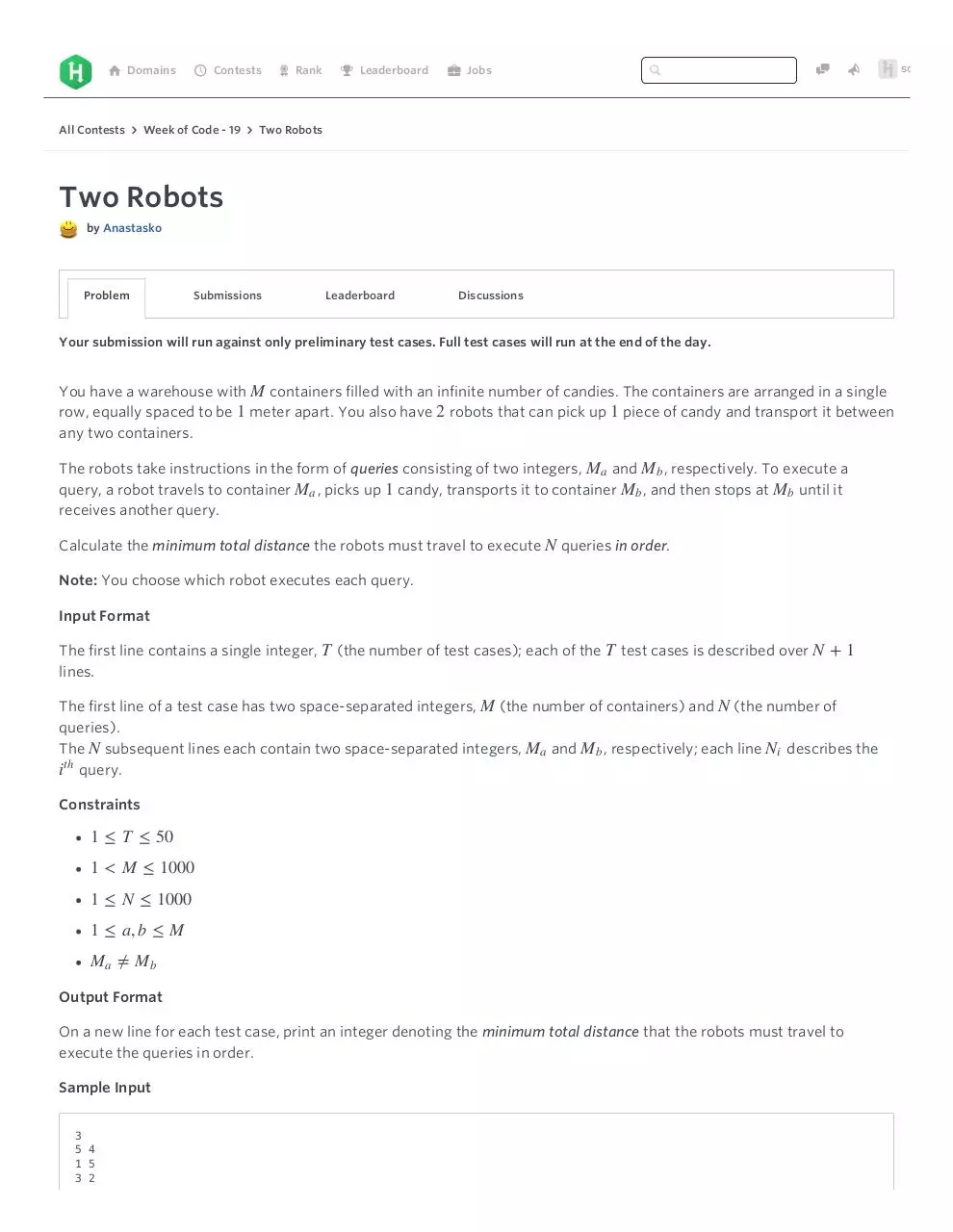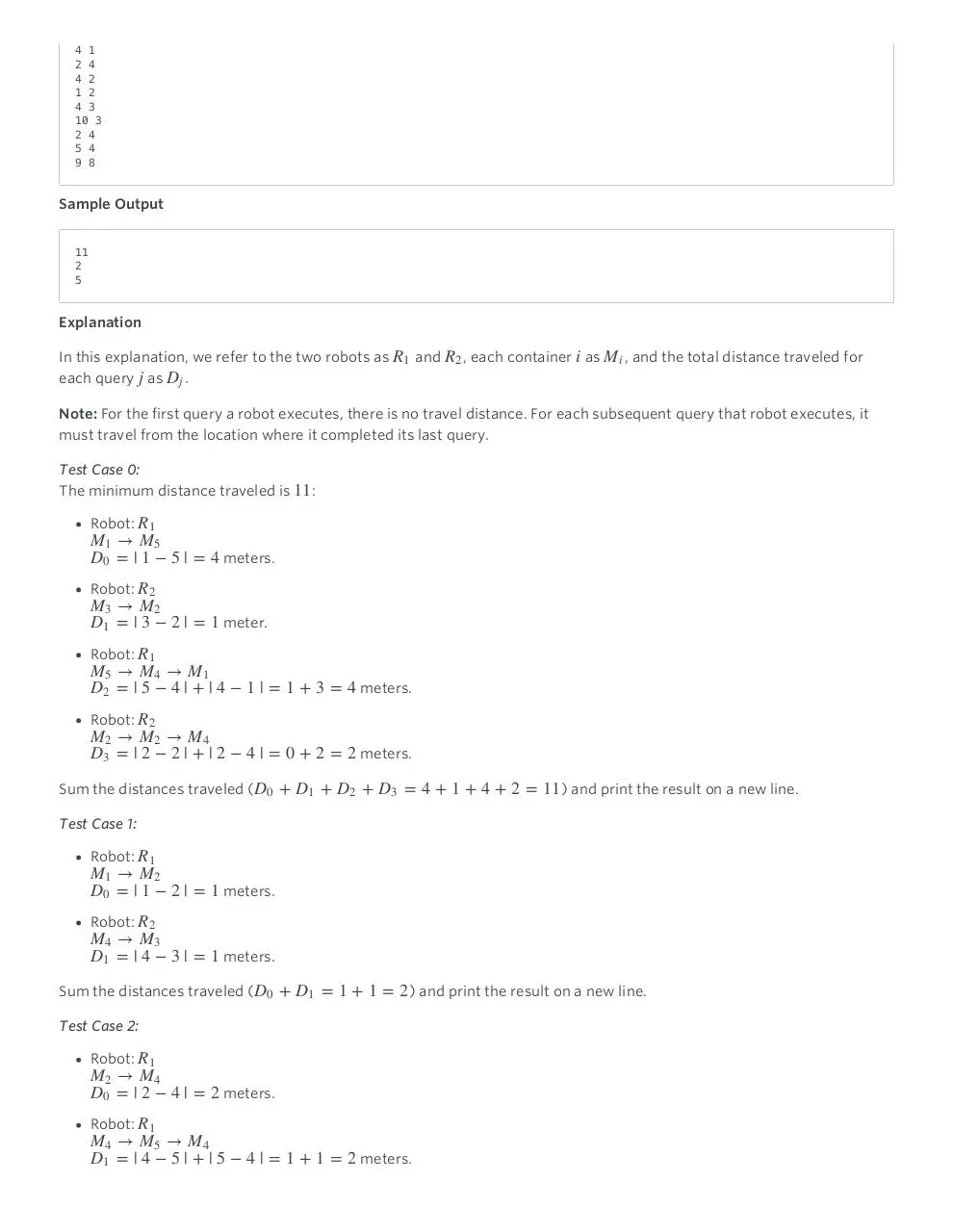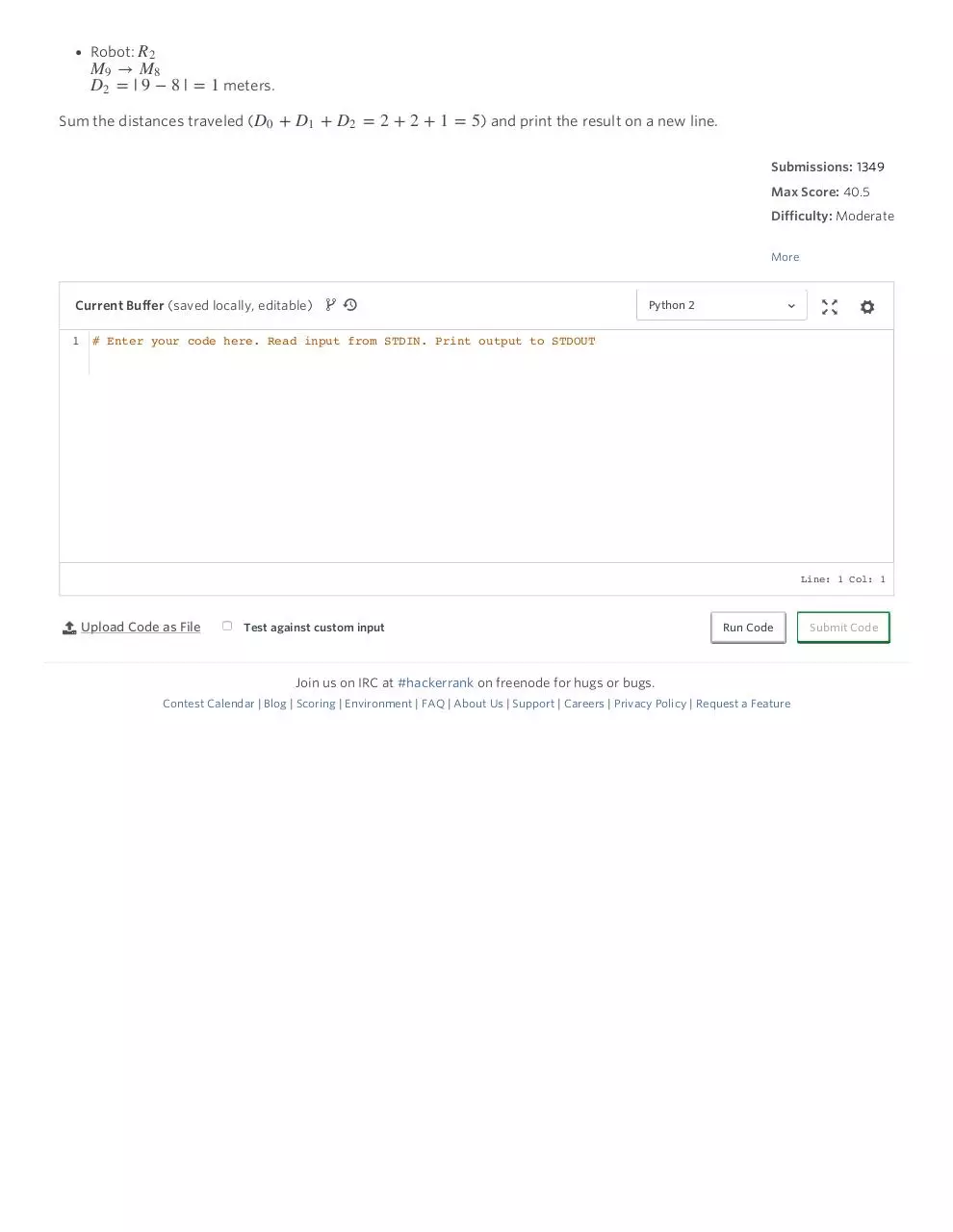# Two Robots Challenge Week of Code 19 HackerRank .pdf

### File information

This PDF 1.4 document has been generated by Mozilla/5.0 (Macintosh; Intel Mac OS X 10_11_3) AppleWebKit/537.36 (KHTML, like Gecko) Chrome/48.0.2564.109 Safari/537.36 / Skia/PDF, and has been sent on pdf-archive.com on 19/02/2016 at 05:37, from IP address 71.231.x.x. The current document download page has been viewed 590 times.
File size: 1.15 MB (3 pages).
Privacy: public file

### Document preview

⌂  Domains

All Contests

  Contests

  Rank

  Jobs

  Week of Code - 19    Two Robots

Two Robots

Problem

Submissions

Discussions

Your submission will run against only preliminary test cases. Full test cases will run at the end of the day.

You have a warehouse with M  containers filled with an infinite number of candies. The containers are arranged in a single
row, equally spaced to be 1  meter apart. You also have 2  robots that can pick up 1  piece of candy and transport it between
any two containers.

The robots take instructions in the form of queries consisting of two integers, M a  and M b , respectively. To execute a
query, a robot travels to container M a , picks up 1  candy, transports it to container M b , and then stops at M b  until it

Calculate the minimum total distance the robots must travel to execute N  queries in order.

Note: You choose which robot executes each query.

Input Format

The first line contains a single integer, T  (the number of test cases); each of the T  test cases is described over N + 1
lines.

The first line of a test case has two space-separated integers, M  (the number of containers) and N  (the number of
queries).
The N  subsequent lines each contain two space-separated integers, M a  and M b , respectively; each line Ni  describes the
th

i

query.

Constraints

1

T

1 &lt; M

1

N

1

a, b

Ma

50

1000
1000

M

Mb

Output Format

On a new line for each test case, print an integer denoting the minimum total distance that the robots must travel to
execute the queries in order.

Sample Input

3
54
15
32

someknow

41
24
42
12
43
10 3
24
54
98

Sample Output

11
2
5

Explanation

In this explanation, we refer to the two robots as R 1  and R 2 , each container i  as M i , and the total distance traveled for
each query j  as Dj .

Note: For the first query a robot executes, there is no travel distance. For each subsequent query that robot executes, it

must travel from the location where it completed its last query.

Test Case 0:

The minimum distance traveled is 11 :

Robot: R 1

M1

M 5

D0 = | 1

− 5 |

= 4  meters.

Robot: R 2

M3

M 2

D1 = | 3

− 2 |

Robot: R 1

M5

M4

D2 = | 5

M2

D3 = | 2

M 1

− 4 | + | 4 − 1 |

Robot: R 2

M2

= 1  meter.

= 1 + 3 = 4  meters.

M 4

− 2 | + | 2 − 4 |

= 0 + 2 = 2  meters.

Sum the distances traveled (D0 + D1 + D2 + D3 = 4 + 1 + 4 + 2 = 11 ) and print the result on a new line.

Test Case 1:

Robot: R 1

M1

M 2

D0 = | 1

− 2 |

= 1  meters.

Robot: R 2

M4

M 3

D1 = | 4

− 3 |

= 1  meters.

Sum the distances traveled (D0 + D1 = 1 + 1 = 2 ) and print the result on a new line.

Test Case 2:

Robot: R 1

M2

M 4

D0 = | 2

− 4 |

Robot: R 1

M4

M5

D1 = | 4

R

= 2  meters.

M 4

− 5 | + | 5 − 4 |

= 1 + 1 = 2  meters.

Robot: R 2

M9

M 8

D2 = | 9

− 8 |

= 1  meters.

Sum the distances traveled (D0 + D1 + D2 = 2 + 2 + 1 = 5 ) and print the result on a new line.

Submissions: 1349

Max Score: 40.5

Difficulty: Moderate

More

  

Current Buffer (saved locally, editable)

Python 2

1 # Enter your code here. Read input from STDIN. Print output to STDOUT

Line: 1 Col: 1

Test against custom input

Run Code

Join us on IRC at #hackerrank on freenode for hugs or bugs.
Contest Calendar | Blog | Scoring | Environment | FAQ | About Us | Support | Careers | Privacy Policy | Request a Feature

Submit CodeTwo Robots _ Challenge _ Week of Code - 19 _ HackerRank.pdf (PDF, 1.15 MB)

### Share on social networks

#### HTML Code

Copy the following HTML code to share your document on a Website or Blog